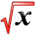# Derivatives of logarithms and exponentialsThis article is part of the MathHelp Tutoring Wiki

General formula:

$d/dx(lnx)=1/x$$d/dx(e^{x})=e^{x}$Examples:

Q: Find $f'(x)$of $f(x)=x^{3}+lnx^{5}$A:

f'(x)=d/dx(x3+ln x5)

=d/dx(x3)+d/dx(ln x5) =3x2+5/x

Note: d/dx(ln x5)=5/x

General Formula:

d/dx(ecx)= cecx

Example:

Q1: find f'(x),if

(1) f(x)=5e-2x

(2) f(x)=e-x/5

A1:

(1) f'(x)=d/dx (5e-2x)

=5d/dx (e-2x)

=5*(-2)e-2x

=-10e-2x

```(2) f'(x)=d/dx(e-5/x)
```

=-1/5 e-5/x

Q2: find f'(x) if f(x)=3x

A2:

f'(x)=d/dx(3x)

=d/dx(eln3x)

= d/dx(exln3)

=ln3 * exln3

=ln3* 3x

General formula:

```d/dx(bx)= (lnb)*bx

**Example:**
```

Q1:if at some time you have \$2000 in an account paying an interest rate of 6% (continuous compounding), what is the instantaneous rate of change in your money account?

Recall: A= Pert

dA/dt= d/dt(Prt)= P d/dt(ert)=Prert

=r A(t)

=2000*6%=120

Q2: Find the point where the graph of y=3e5x+2 has a slope of 5

= 3d/dx(e5x)+0

=15e5x+0

=15 e5x

To find the point we set

dy/dx =5= 15e5x

e5x=1/3

ln(e5x)=ln(1/3)

5x= -ln3

x=(-1/5)*ln3

The y coordinate is y=3eln(1/3)/5+2

=3eln(1/3)+2

=3*1/3+2=3

So the point is ((-1/5)ln3,3)